# SSAT Elementary Level Math : How to find the perimeter of a triangle

## Example Questions

1 2 4 Next →

### Example Question #31 : How To Find The Perimeter Of A Triangle

Find the perimeter of the triangle shown below: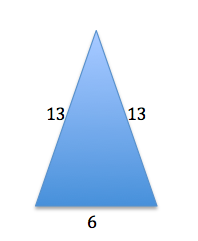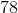Explanation:

The perimeter is the sides added together.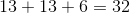### Example Question #32 : How To Find The Perimeter Of A Triangle

Use the following to answer the question.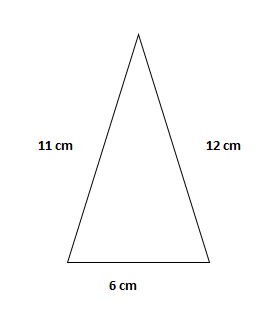Find the perimeter of the triangle.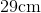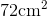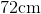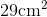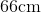Explanation:

To find the perimeter of a triangle, we will use the following formula: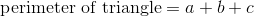where a, b, and c are the lengths of the sides of the triangle.

So, in the trianglewe can see the lengths of the sides are 11cm, 12cm, and 6cm.  Knowing this, we can substitute into the formula.  We get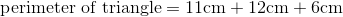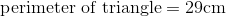### Example Question #33 : How To Find The Perimeter Of A Triangle

Find the perimeter of an equilateral triangle with a base of 16in.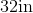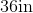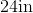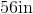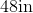Explanation:

To find the perimeter of a triangle, we will use the following formula:where a, b, and c are the lengths of the sides of the triangle.

Now, we know the base of the triangle has a length of 16in.  Because it is an equilateral triangle, all lengths are the same.  Therefore, all lengths are 16in.

Knowing this, we can substitute into the formula.  We get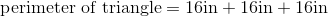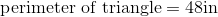### Example Question #34 : How To Find The Perimeter Of A Triangle

The perimeter of an equilateral triangle is 27in.  Find the length of one side of the triangle.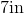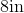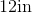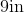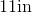Explanation:

To find the perimeter of an equilateral triangle, we will use this formula: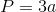where a is the length of one side.  Now, to find the length of one side, we will solve for a

We know the perimeter of the triangle is 27in.  So, we will substitute.  We get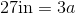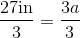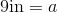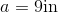Therefore, the length of one side of the triangle is 9in.

### Example Question #35 : How To Find The Perimeter Of A Triangle

Use the following triangle to solve the problem:Find the perimeter.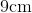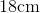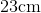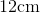Explanation:

To find the perimeter of a triangle, we will use the following formula: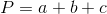where a, b, and c are the lengths of the sides of the triangle.

Now, given the trianglewe can see it has sides of length 11cm, 6cm, and 12cm.  So, we can substitute.  We get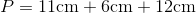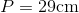1 2 4 Next →